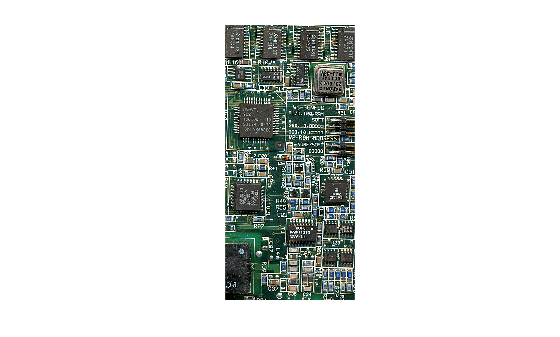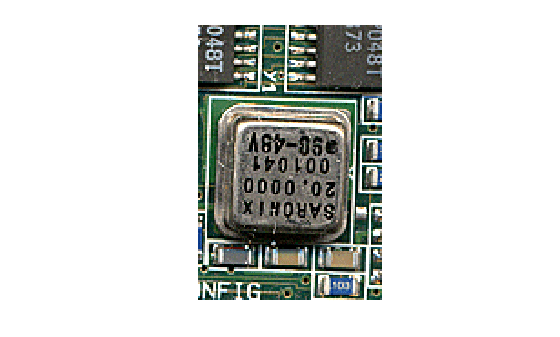# Rectangle

Spatial extents of 2-D rectangular region

Since R2019b

## Description

A `Rectangle` object stores the spatial extents of a 2-D rectangular region.

## Creation

You can create a `Rectangle` object in these ways.

• `centerCropWindow2d` — Create a `Rectangle` of a specified size whose position is centered on an image of different size.

• `randomWindow2d` — Create a `Rectangle` whose position is selected randomly from within an image of different size. You can specify the size of the rectangle or a range of valid aspect ratios and relative areas of the rectangle.

• Running the command

`r = images.spatialref.Rectangle(XLimits,YLimits)`
creates a `Rectangle` object and sets the `XLimits` and `YLimits` properties.

## Properties

expand all

Minimum and maximum limits of the x-axis, specified as a 2-element numeric vector of the form `[min max]`, where `max` is greater than `min`.

Data Types: `single` | `double` | `int8` | `int16` | `int32` | `int64` | `uint8` | `uint16` | `uint32` | `uint64`

Minimum and maximum limits of the y-axis, specified as a 2-element numeric vector of the form `[min max]`, where `max` is greater than `min`.

Data Types: `single` | `double` | `int8` | `int16` | `int32` | `int64` | `uint8` | `uint16` | `uint32` | `uint64`

## Examples

collapse all

```I = imread('parkavenue.jpg'); imshow(I)```Specify a target window size as a two-element vector of the form [width, height].

`targetSize = [300 600];`

Create a `Rectangle` object that specifies the spatial extent of the crop window.

`r = centerCropWindow2d(size(I),targetSize);`

Crop the image to the spatial extents. Display the cropped region.

```J = imcrop(I,r); imshow(J)``````I = imread('board.tif'); imshow(I)```Create a `Rectangle` object by specifying the horizontal and vertical spatial extents of the cropping window.

`r = images.spatialref.Rectangle([200 300],[50 200])`
```r = Rectangle with properties: XLimits: [200 300] YLimits: [50 200] ```

Crop the image to the spatial extents. Display the cropped region.

```J = imcrop(I,r); imshow(J)```## Version History

Introduced in R2019b